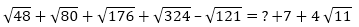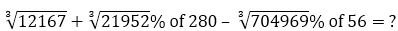SBI PO Quantitative Aptitude Quiz

Here, we are providing SBI PO Mains Study plan as there is left not enough time to deal in details. The questions asked in the quantitative aptitude section are calculative and very time-consuming. But once dealt with proper strategy, speed, and accuracy, this section can get you the maximum marks in the examination. Following is the Quantitative Aptitude quiz to help you practice with the best of latest pattern questions
For Queries related to Adda247 Ultimate for IBPS RRB 2019 Prelims, Click Here or mail us at ultimate@adda247.com

Directions (1-5): Pie chart given below shows expenditure of six persons and table given below shows ratio between expenditure on three different products by each person. Study the data carefully and answer the following questions.
Note- Expenditure is considered for food, rent and transportation only.

Q1. Find the difference between expenditure of B on Food to that of C’s on food if expenditure of ‘F’ on Food is Rs. 6750.
Rs. 2880
Rs. 3060
Rs. 3240
Rs. 3420
Rs. 3600

Q2. A’s expenditure on transport is what percent more than E’s expenditure on transport?
10%
15%
20%
25%
30%

Q3. Find the average expenditure of A, B and C together on transport if average expenditure of D, E and F together on transport is Rs. 4860.
Rs. 8955
Rs. 9090
Rs. 9225
Rs. 9360
Rs. 9495

Q4. Expenditure of B and C on Rent is how much more than expenditure of D and E on Rent if expenditure of F on Rent is Rs. 8100.
Rs. 2556
Rs. 2664
Rs. 2772
Rs. 2880
Rs. 2988

Q5. If A’s expenditure on rent is Rs. 8100, then find total expenditure of all six persons together? (in lakh)
1.53
1.62
1.44
1.35
1.08

Directions (6-10): What should come in place of the question mark (?) in the following question?

Q6.1627.26
1842.28
2159.51
1972.88
2171.99

Q7.4(√5+√7)
6(√3+√5)
4(√3+√5)
3(√5+√7)
9(√2+√3)

Q8.8517
9517
8717
7087
9087

Q9.41.56
51.46
51.56
65.56
71.56

Q10.14
19
12
15
None of these

Q11. A and B started a business in partnership with the investment of Rs. 27000 and Rs. 36000 respectively, after 4 month A withdraw 5000 Rs. and B added 6000 Rs. more and C joined with 35000 Rs. if after one year they get a total profit of Rs. 130500, then find the profit share of C?
Rs. 36000
Rs. 32000
Rs. 35000
Rs. 38000
Rs. 42000

Q12. Sheetal and Sakshi two working employee of Add 247 got salaries per month Rs 24000 and Rs 32000 respectively. Both invest 50% and 40% of their salaries in mutual funds and after a year they got a total profit from their investment as Rs 12400, then find the profit of Sakshi obtained from mutual funds.
Rs.5400
Rs.7200
Rs.9600
Rs.6400
Rs.8400

Q13. P being a working partner gets 10% of the profit as salary, the remaining is shared between P, Q, R in the ratio 2 : 3 : 4, If P gets Rs. 300000, find the share of Q.
Rs.200,000
Rs.300,000
Rs.400,000
Rs.500,000
Rs.550,000

Q14. In a set of prime and composite numbers, the composite numbers are twice the number of prime numbers and the average of all the numbers of the set is 9. If the number of prime numbers and composite numbers are exchanged then the average of the set of numbers is increased by 2 and during the exchange of the numbers the average of the prime numbers and composite numbers individually remained constant, then find the ratio of the average of composite numbers to the average of prime numbers (initially).
7 : 13
13 : 7
9 : 11
13 : 15
Can’t be determined

Q15. Ages of A, B, and C are in geometric progression while ages of A, Q and R is in arithmetic progression. If ratio between common difference of arithmetic progression formed by second group (A, Q, R) and common ratio of geometric progression formed by first group (A, B and C) is 2: 1 and total sum of ages of first group (A, B, C) is 182 and total sum of second group (A, Q, R) is 60 then which of the following options given below will be the ages of A, B, C respectively.
126, 42, 14
42, 14, 126
14, 42, 126
36, 60, 86
Can’t be determined

You May also like to Read: